# Year 3 Maths Printable Worksheets

Our fun and engaging maths worksheets cover all areas of the Y3 maths curriculum, including counting, mental maths, solving problems and calculations, shape and space, multiplication tables, number facts, measuring, data handling, and much more. Whether it's finding the perimeter of 2D shapes or using the 24-hour clock, you will be sure to find a year 3 maths worksheet to suit your child's needs.

Filters

### Hundreds, tens and units 2

This year 3 maths worksheet asks students to read the number shown on each abacus.

Age: 7 - 8
1
2
3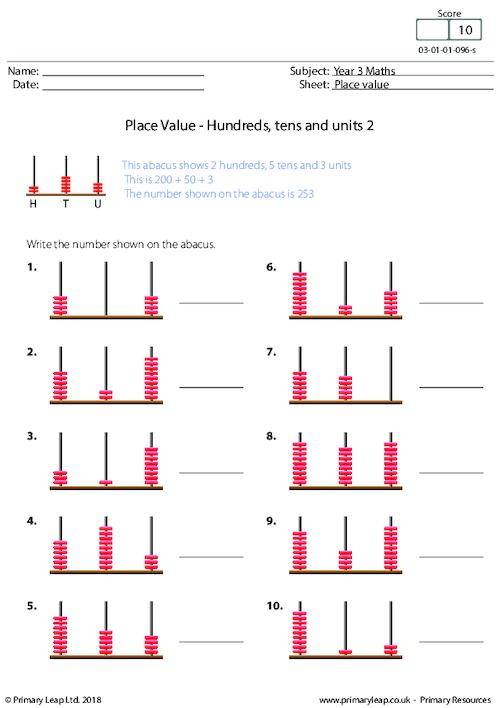### Hundreds, tens and units 1

This KS2 maths resource asks students to read the number shown on each abacus.

Age: 7 - 8
1
2
3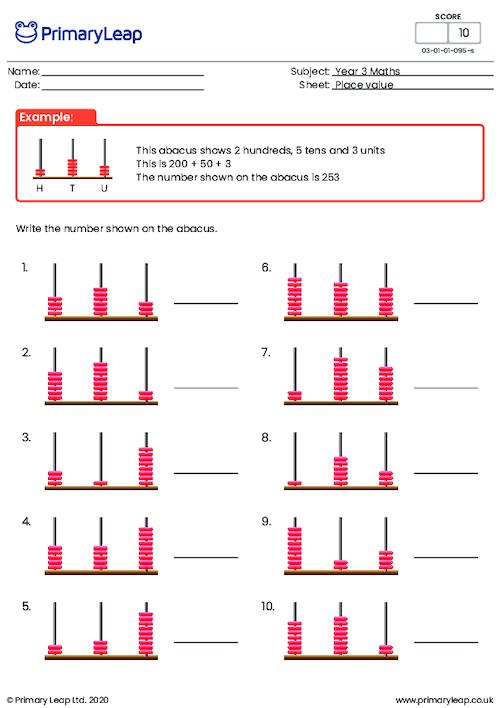### Find the missing sign

A KS2 maths resource on finding the missing sign to complete the sum.

Age: 7 - 8
1
2
3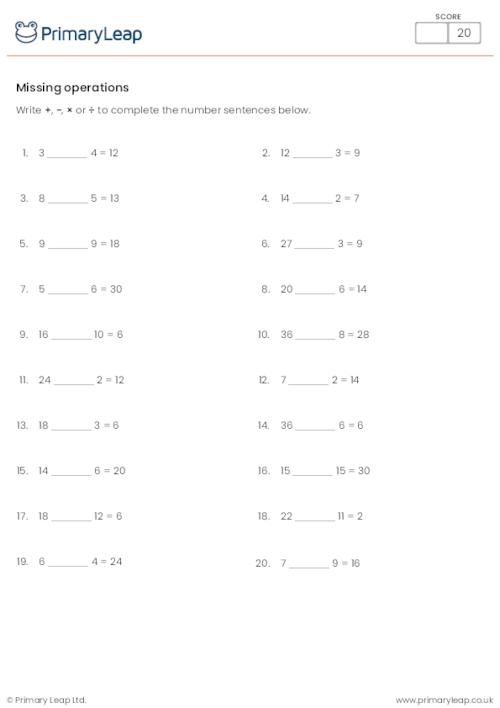### Multiplying and Dividing 3

A KS2 numeracy resource on multiplication and division - Comparing multiplication and division by 3.

Age: 7 - 8
1
2
3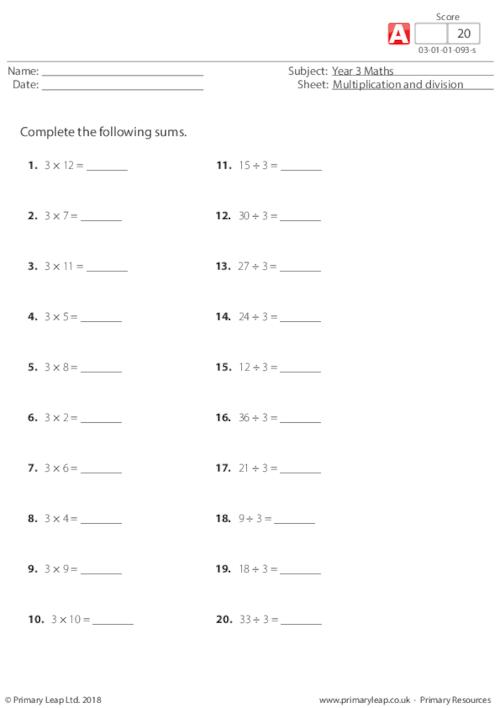### Multiplying and Dividing 2

A KS2 numeracy resource on multiplication and division - Comparing multiplication and division by 5.

Age: 7 - 8
1
2
3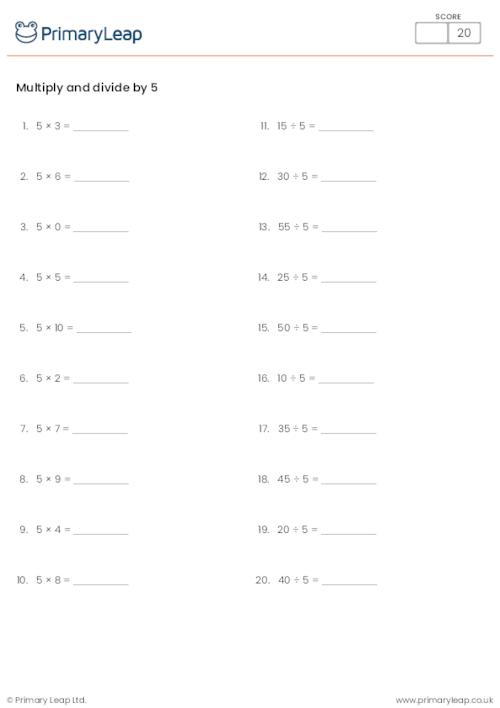### 10 and counting

A KS2 maths resource on counting in 10s.

Age: 7 - 8
1
2
3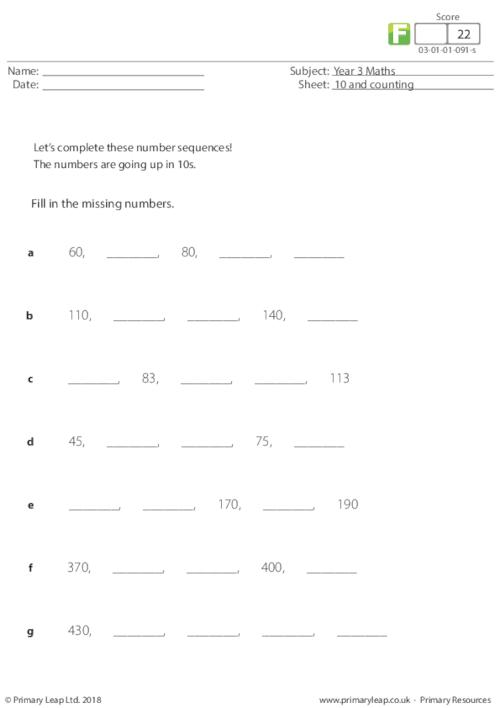### 20 and counting

A year 3 maths resource on counting in 20s.

Age: 7 - 8
1
2
3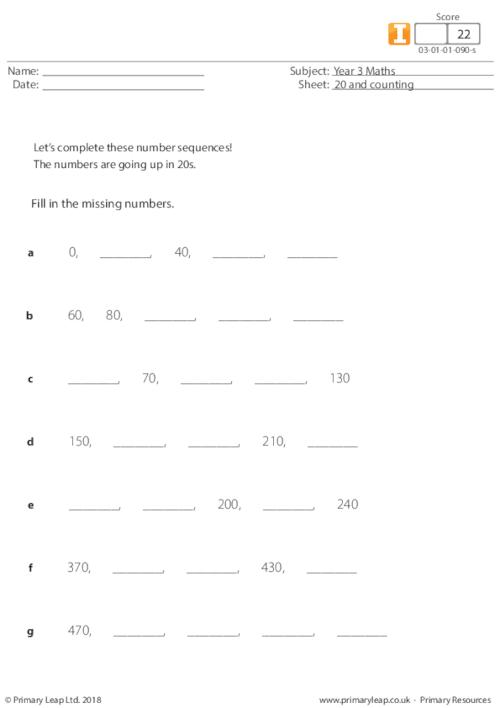### Decimals - Tenths

This year 3 maths resource focuses on decimals and fractions. Students are asked to firstly write the decimal and then the fraction that is being shown by each square.

Age: 7 - 8
1
2
3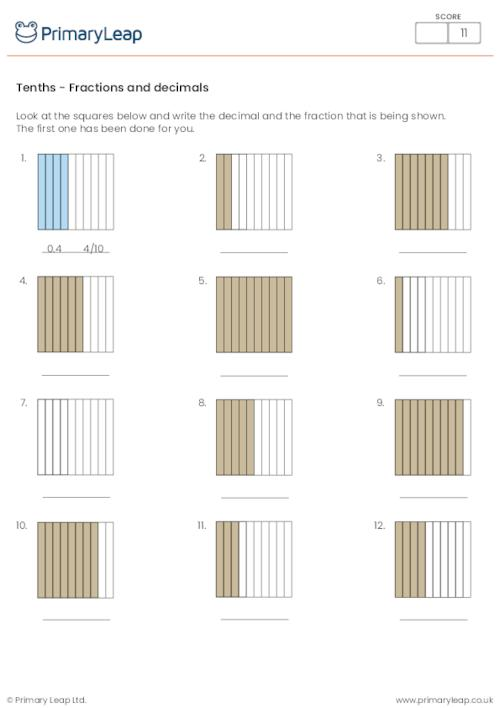### 30 and counting

Year 3 maths printable - This worksheet includes number sequences.

Age: 7 - 8
1
2
3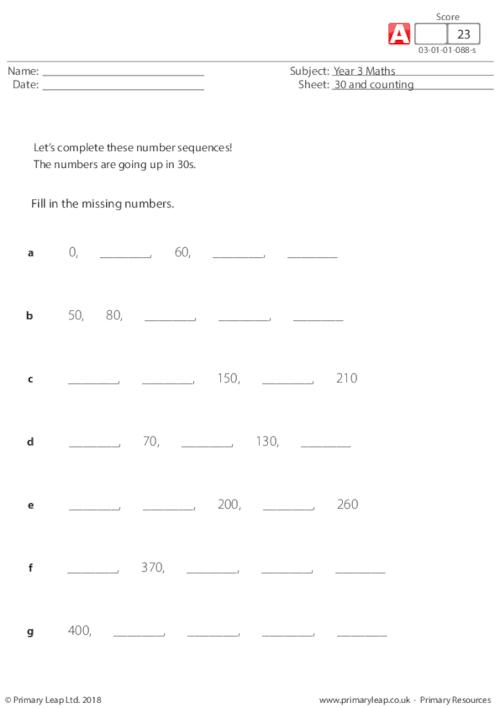### Bonds of 60

Year 3 maths - Bonds of 60. Students find the missing numbers to make 60.

Age: 7 - 8
1
2
3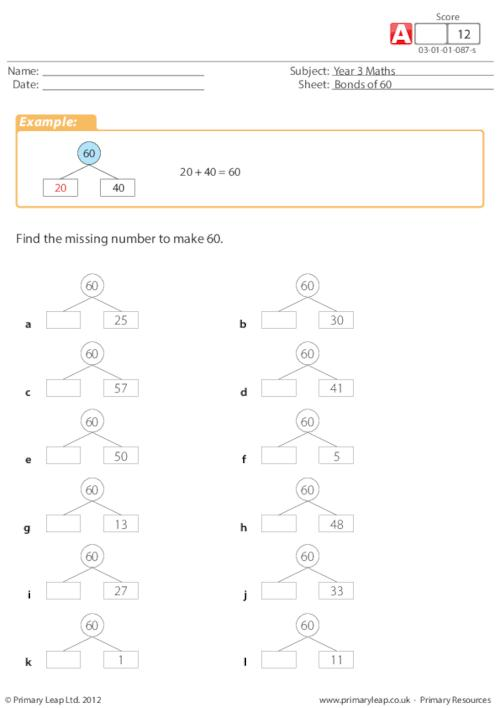### Bonds of 30

A KS2 maths resource on bonds of 30. Students find the missing numbers to make 30.

Age: 7 - 8
1
2
3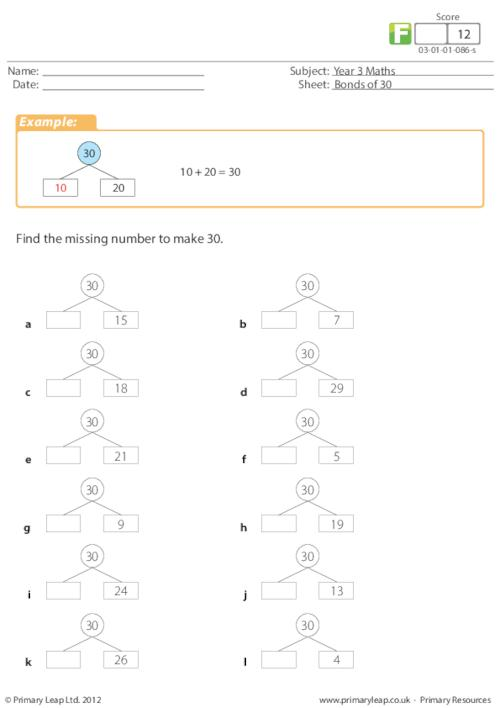### Bonds of 40

Year 3 maths - Students must find the missing number to make 40.

Age: 7 - 8
1
2
3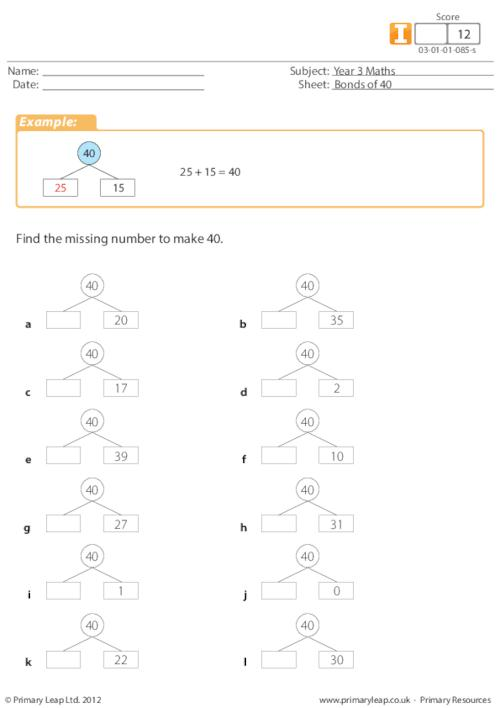Year 3 Maths - Money addition. This worksheet includes 18 money addition sums.

Age: 7 - 8
1
2
3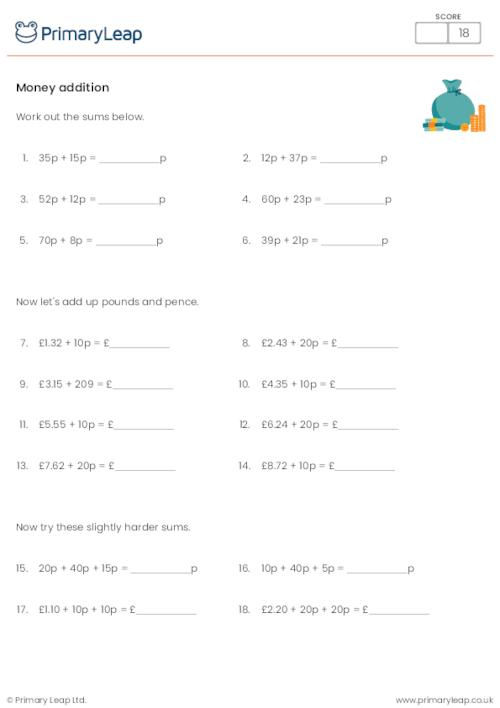### Bonds of 90

A year 3 maths worksheet on finding the missing number to add up to 90.

Age: 7 - 8
1
2
3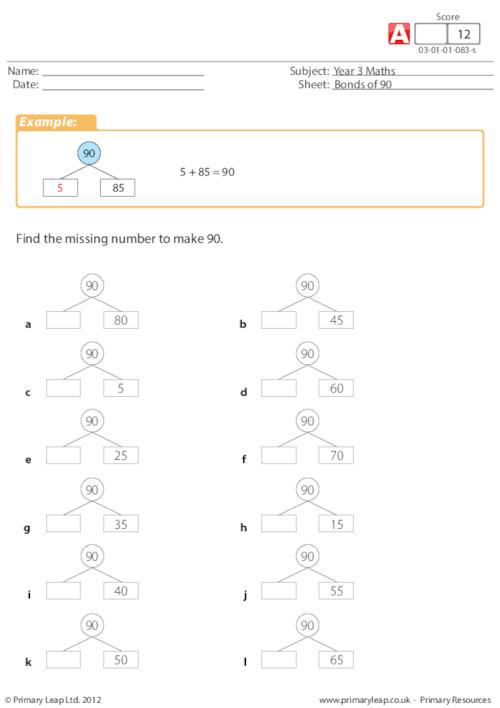### Bonds of 70

A KS2 maths resource on find the missing number to add up to 70.

Age: 7 - 8
1
2
3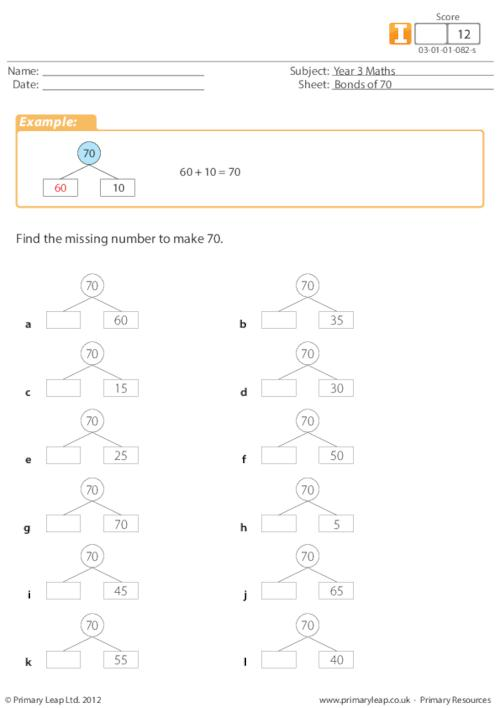### Bonds of 50

A year 3 maths resource on finding the missing number to add up to 50.

Age: 7 - 8
1
2
3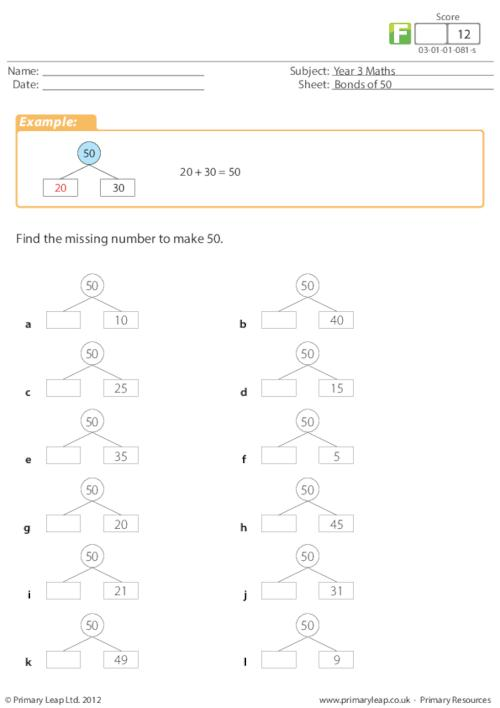### Horizontal Subtraction - Subtracting by 9

This basic maths worksheet for year 3 was created to help kids practise subtracting 9 with horizontal subtraction questions.

Age: 7 - 8
1
2
3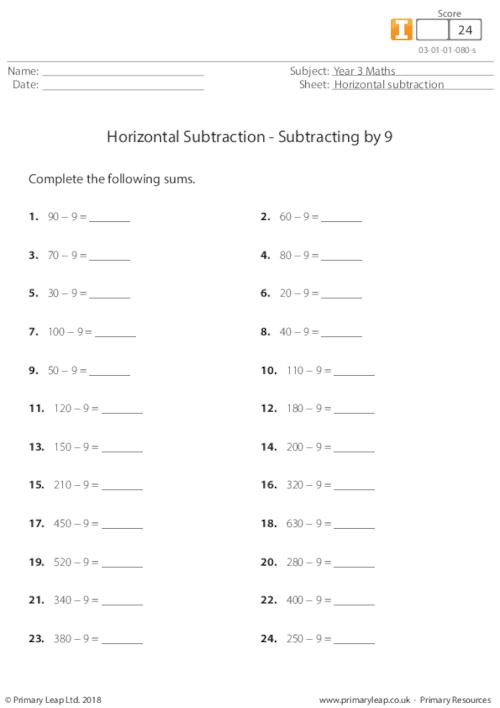### 100 Number square

Year 3 maths worksheet - 100 number square

Age: 7 - 8
1
2
3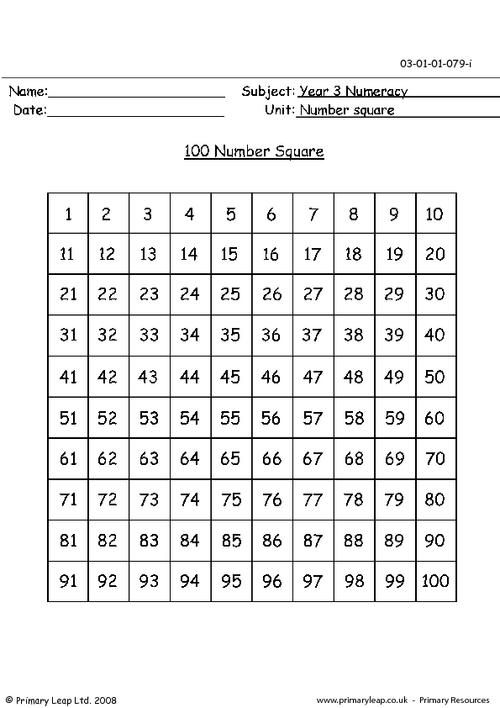### Triangular numbers

Year 3 Math: Triangular numbers - Information sheet.

Age: 7 - 8
1
2
3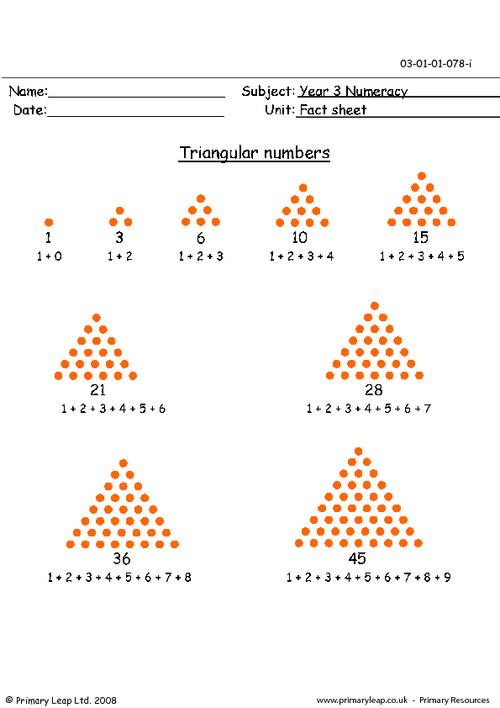### Square numbers

An information sheet for key stage 2 on square numbers and how they are made up.

Age: 7 - 8
1
2
3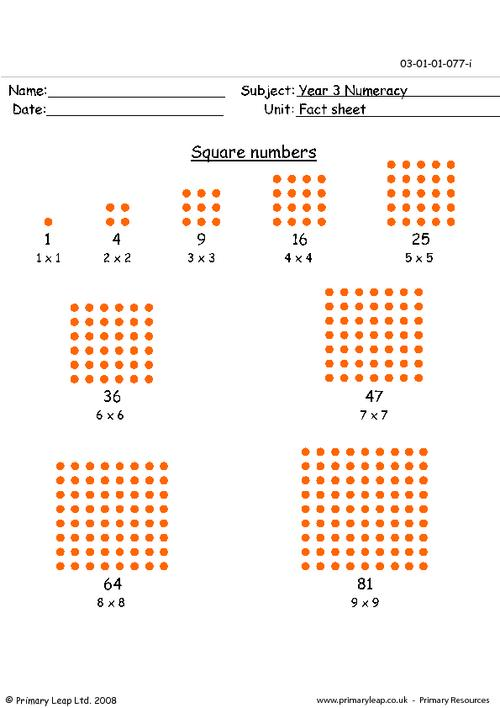### Conversion chart (fraction, decimal, percentage)

Year 3 Math: Conversions - This is an information sheet showing conversions of fractions, decimals and percentages.

Age: 7 - 8
1
2
3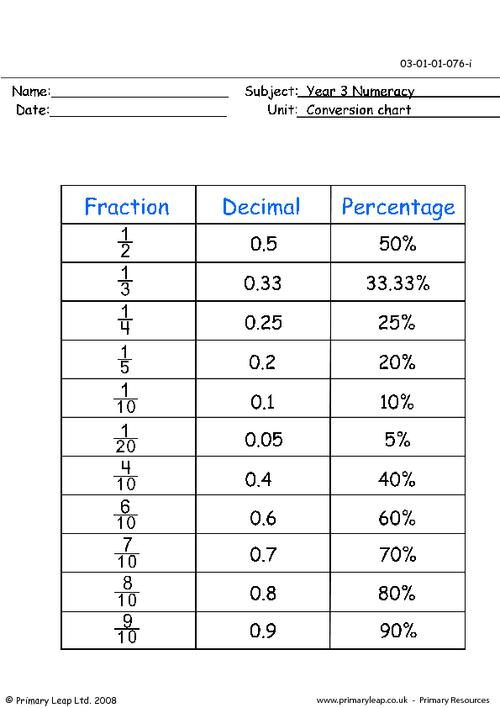### Equivalent Fractions

A KS2 maths resource on equivalent fractions.

Age: 7 - 8
1
2
3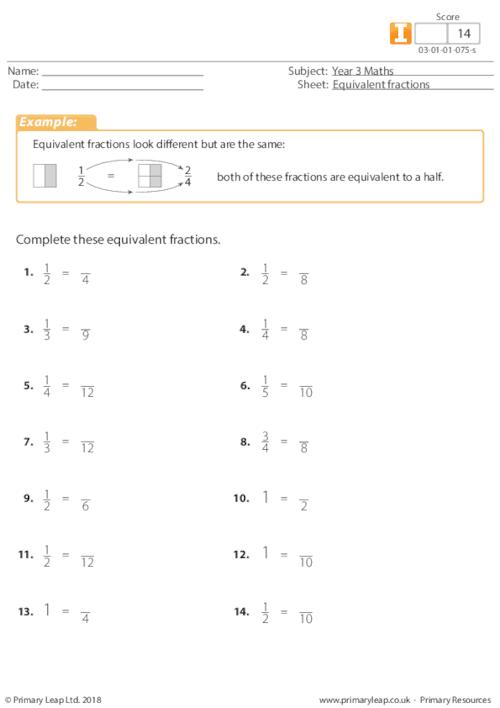### Fractions 3

Halving numbers - A simple KS2 numeracy worksheet where kids find half of the given number.

Age: 7 - 8
1
2
3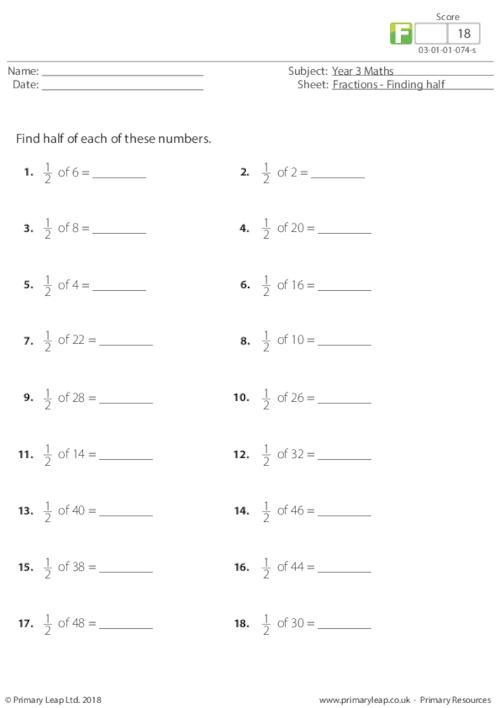### Number fractions

This year 3 numeracy worksheet is great for testing kids for finding fractions of whole numbers.

Age: 7 - 8
1
2
3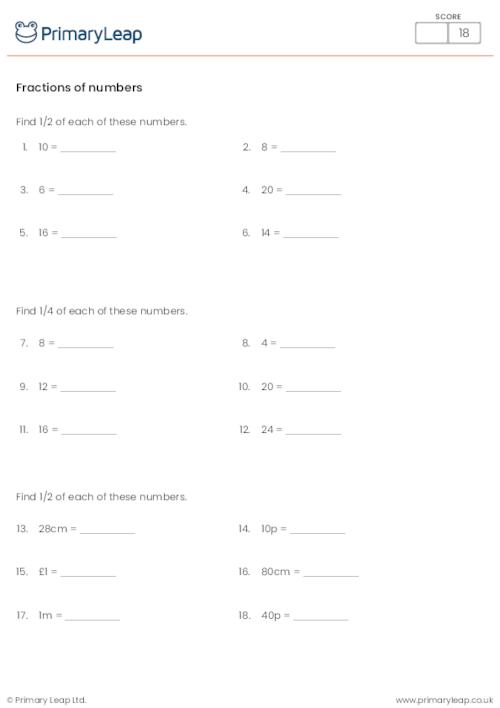### Double and half 2

A useful KS2 resource for children to practise finding half and double of numbers.

Age: 7 - 8
1
2
3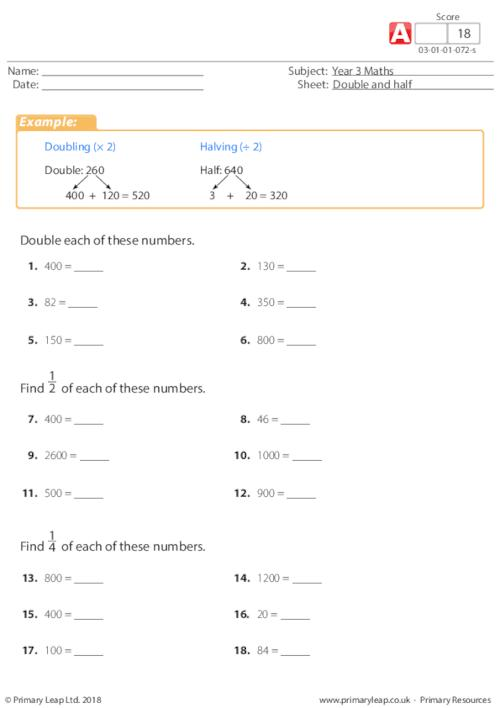### Double and half 1

A KS2 numeracy worksheet on double and half - Find double and half of a number. Numbers up to 1000.

Age: 7 - 8
1
2
3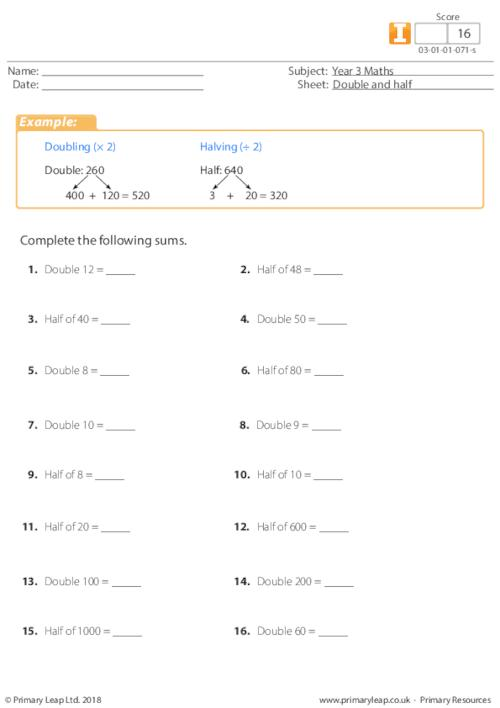### Fractions 2

A KS2 maths resource on fractions.

Age: 7 - 8
1
2
3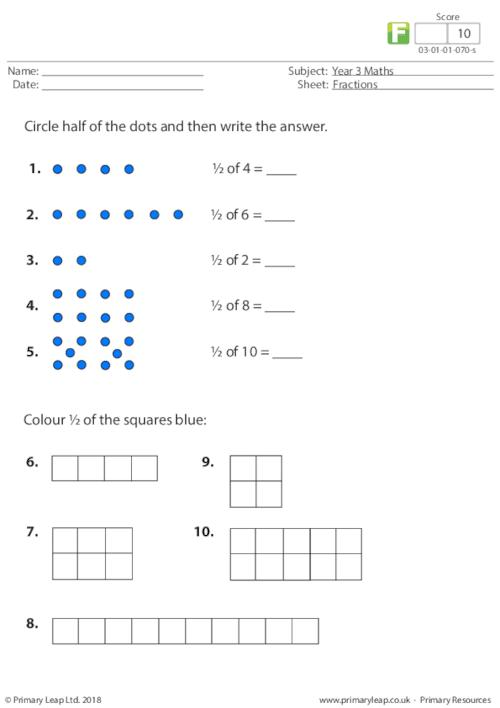### Fractions 1

A year 3 numeracy resource on fractions - Dividing shapes into equal halves.

Age: 7 - 8
1
2
3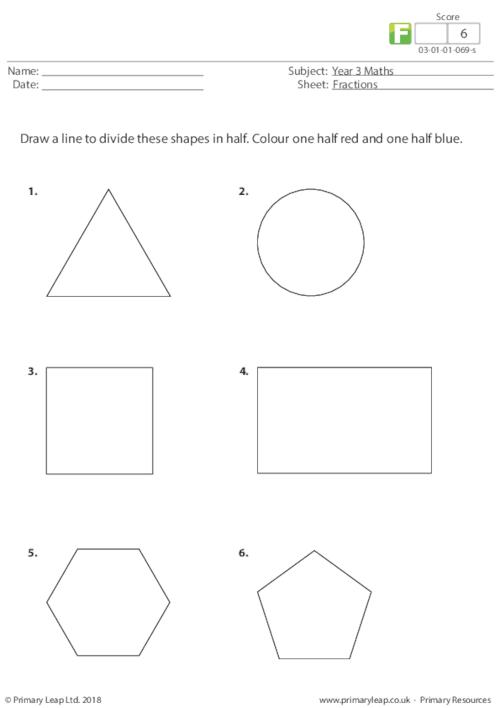### Money 3

This year 3 maths printable is all about adding money amounts. There are 10 questions to answer.

Age: 7 - 8
1
2
3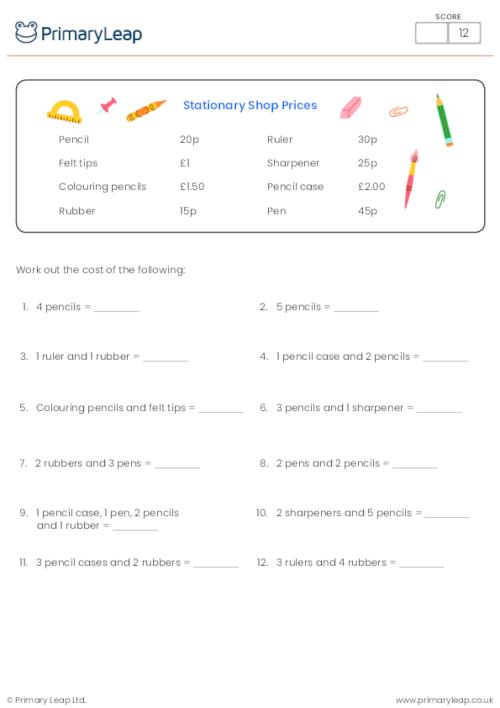### Money 2

Year 3 Numeracy worksheet - Addition of money

Age: 7 - 8
1
2
3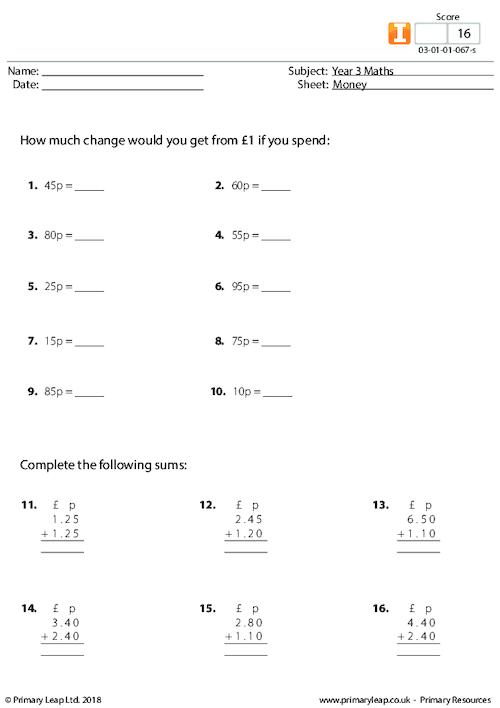### Money 1

A year 3 maths resource about money - Changing pence to pounds and pounds to pence.

Age: 7 - 8
1
2
3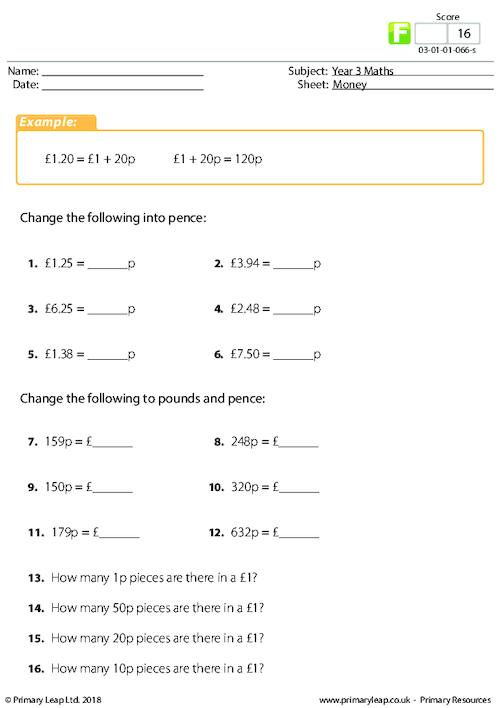### Division 4

A year 3 maths resource on dividing by 2 with remainders.

Age: 7 - 8
1
2
3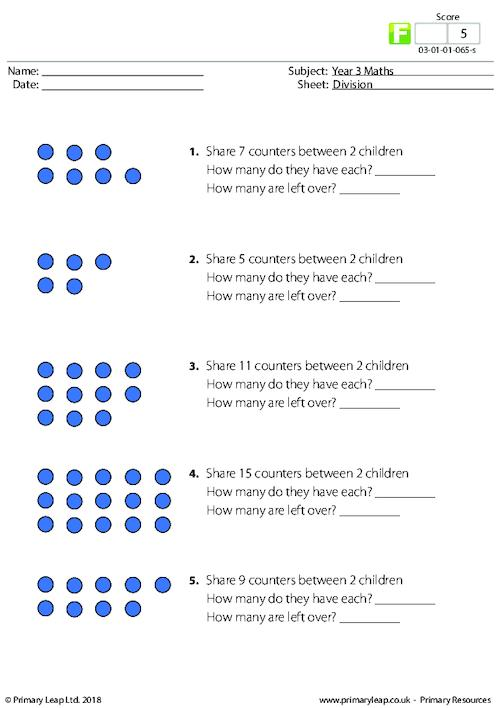### Division 3

A year 3 maths division resource on dividing of 2, 3, 4, and 5 with no remainders.

Age: 7 - 8
1
2
3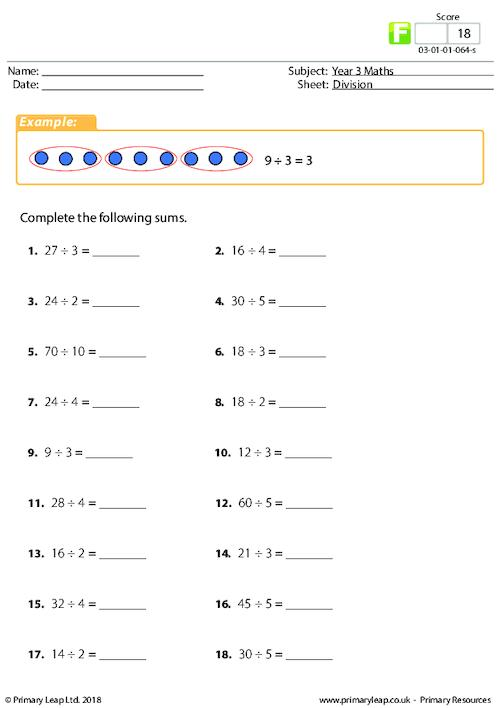### Division 2

A KS2 maths resource on dividing by numbers 2, 3, 4 and 5 with the use of remainders.

Age: 7 - 8
1
2
3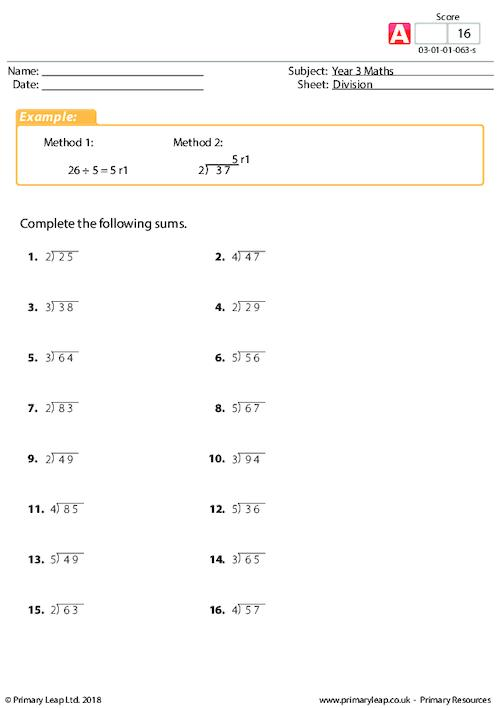### Division 1

A KS2 maths worksheet on dividing numbers by 2, 3, 4 and 5 with no remainders.

Age: 7 - 8
1
2
3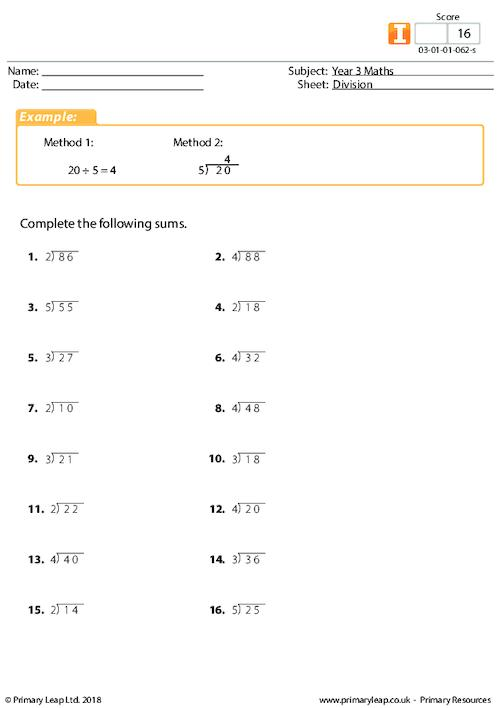### Multiplying and Dividing

A KS2 numeracy resource on multiplication and division - Comparing multiplication and division by 2.

Age: 7 - 8
1
2
3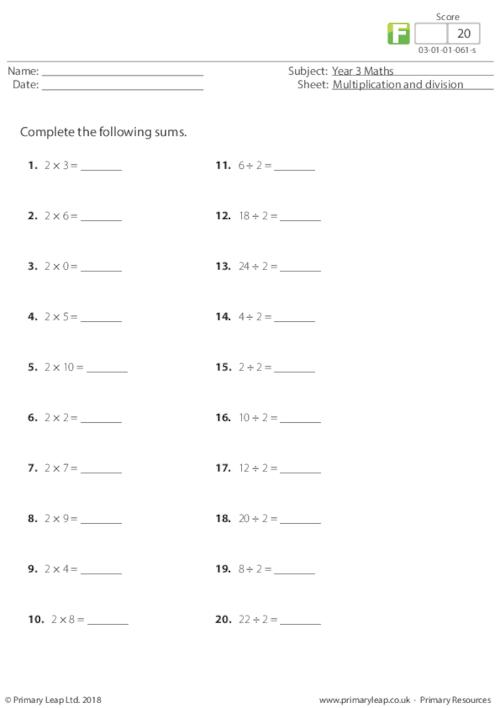### Multiplication

A KS2 maths resource on finding the missing numbers when multiplying by 10 and 100.

Age: 7 - 8
1
2
3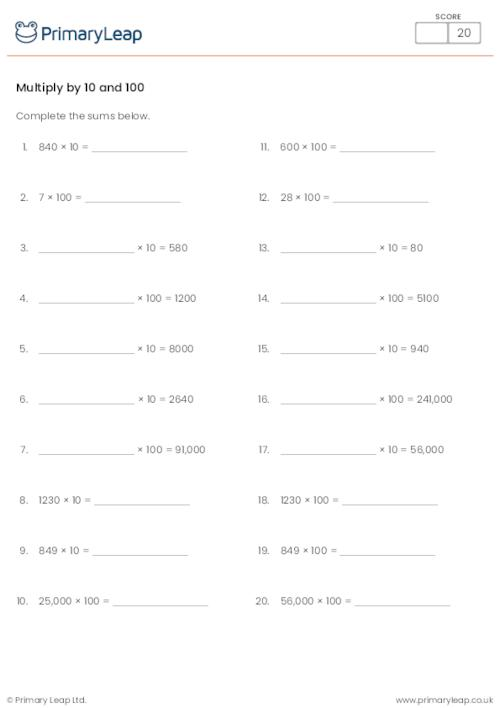### Multiplying tens

A year 3 maths resource on multiplication - Fill in the missing numbers, multiplying by tens.

Age: 7 - 8
1
2
3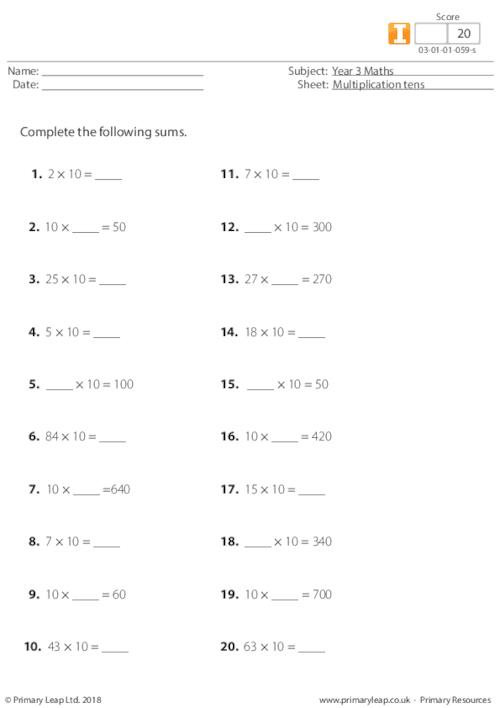### Multiplying by 10

This KS2 worksheet includes horizontal multiplication sums for multiplying by 10.

Age: 7 - 8
1
2
3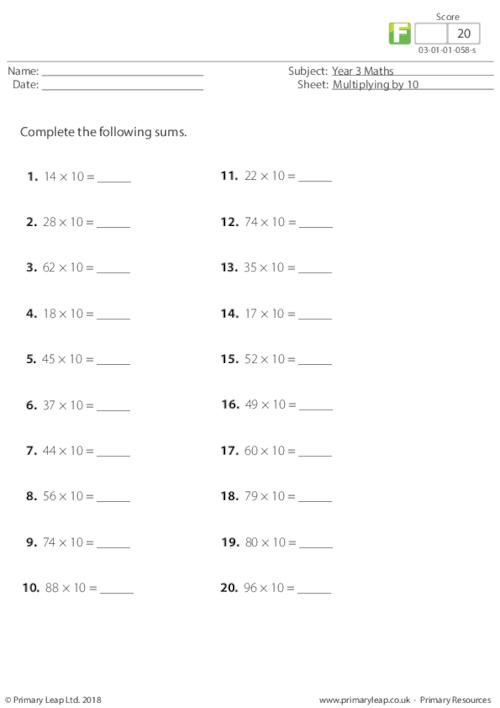### Multiply by 10

A year 3 maths resource including horizontal multiplication sums for multiplying by 10.

Age: 7 - 8
1
2
3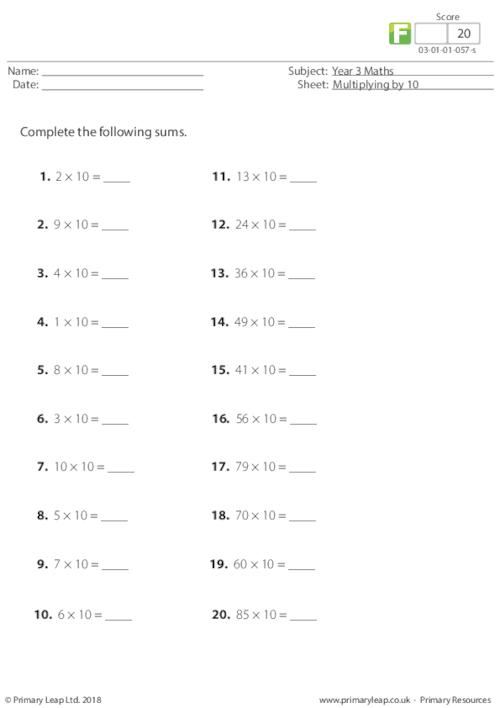### Multiplying in brackets

This year 3 numeracy resource includes horizontal multiplication sums. Don't forget to do the sums in brackets first and then add the answers together.

Age: 7 - 8
1
2
3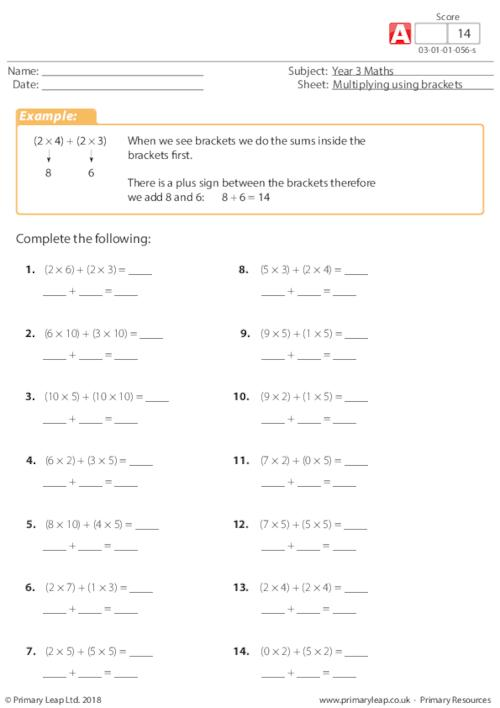### Multiplication twos and tens

A KS2 numeracy resource on doubling larger numbers.

Age: 7 - 8
1
2
3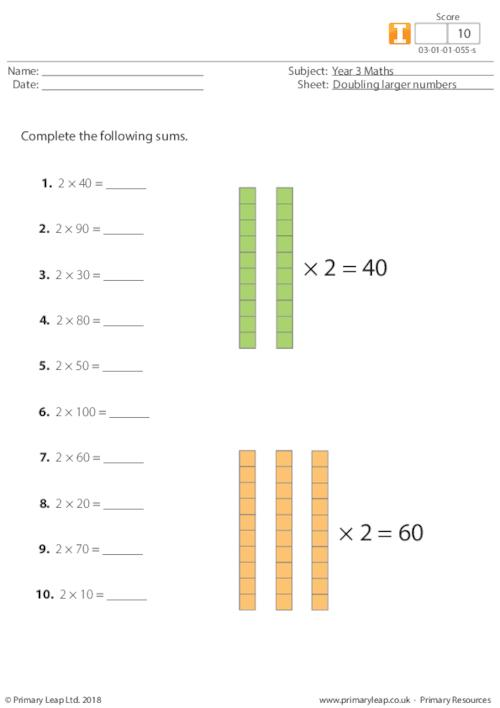### Multiplication

A KS2 numeracy resource including vertical multiplication sums with the numbers 2, 3, 4 and 5.

Age: 7 - 8
1
2
3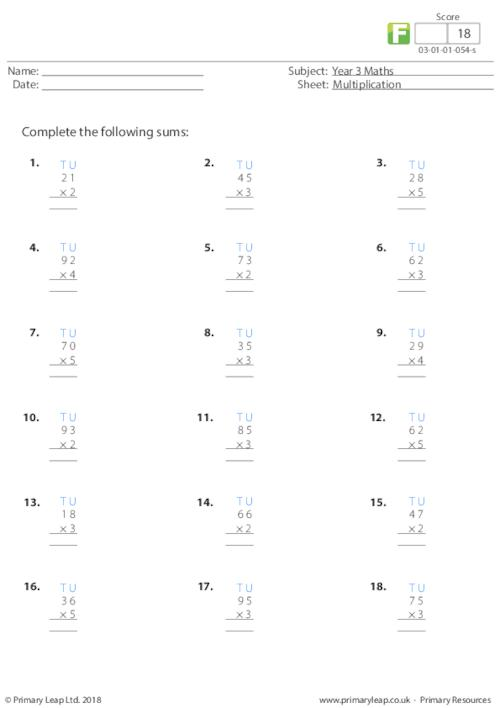### Multiplication vertical x 5

A year 3 maths resource with vertical multiplication sums of 5.

Age: 7 - 8
1
2
3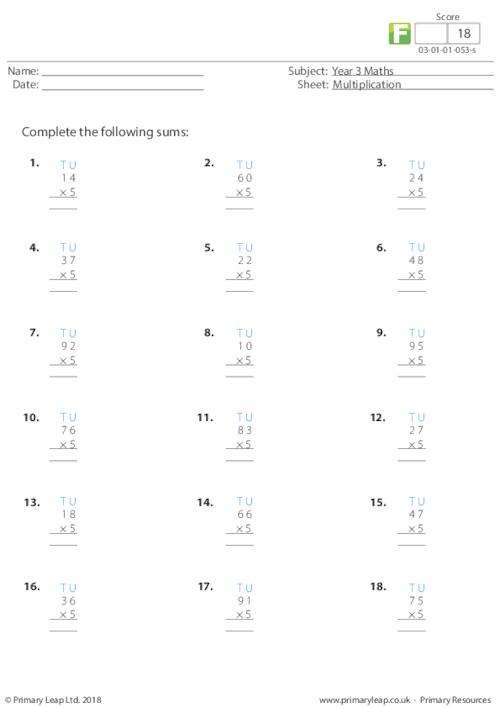### Multiply by 5

A KS2 maths resource on filling in the missing divisible number to complete the horizontal and vertical multiplication sums.

Age: 7 - 8
1
2
3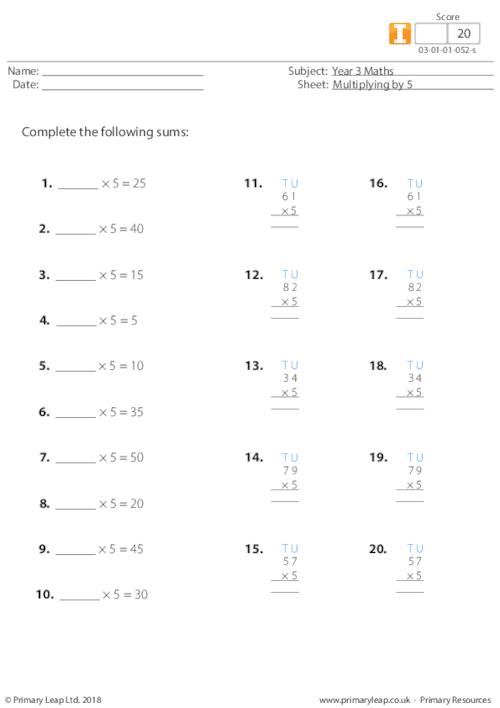### Multiply by 2, 5 and 10

A year 3 maths worksheet on multiplication - This worksheet includes both horizontal and vertical multiplication sums with the numbers 2, 5 and 10.

Age: 7 - 8
1
2
3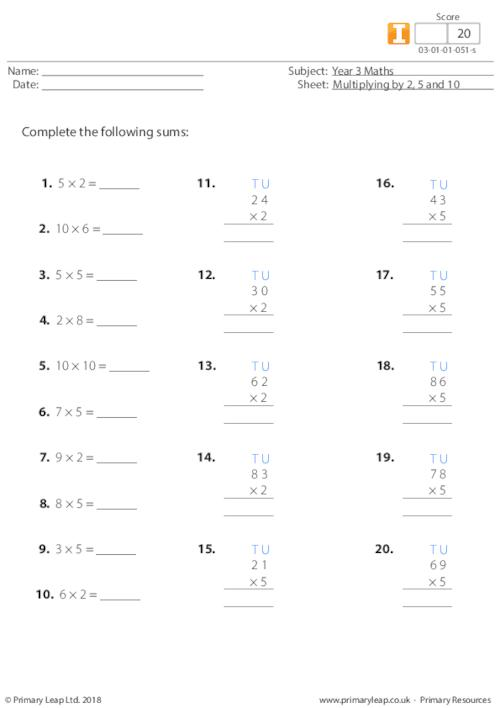### Multiply by 5

A KS2 maths resource on understanding multiples of 5.

Age: 7 - 8
1
2
3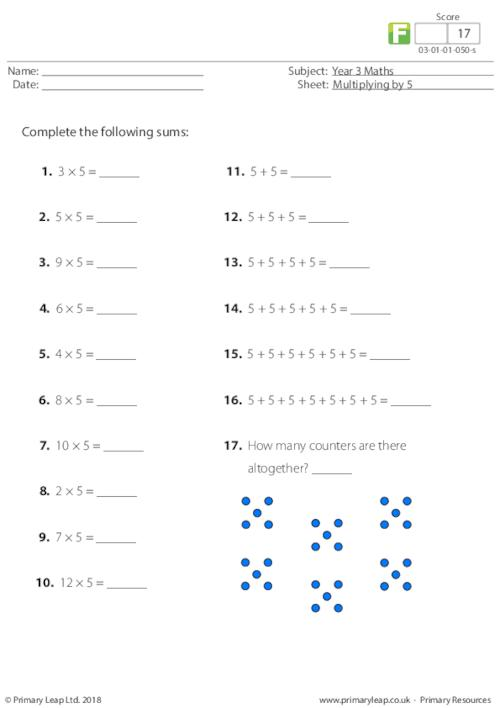### Multiplication x 4

A KS2 numeracy resource on vertical multiplication using the number 4.

Age: 7 - 8
1
2
3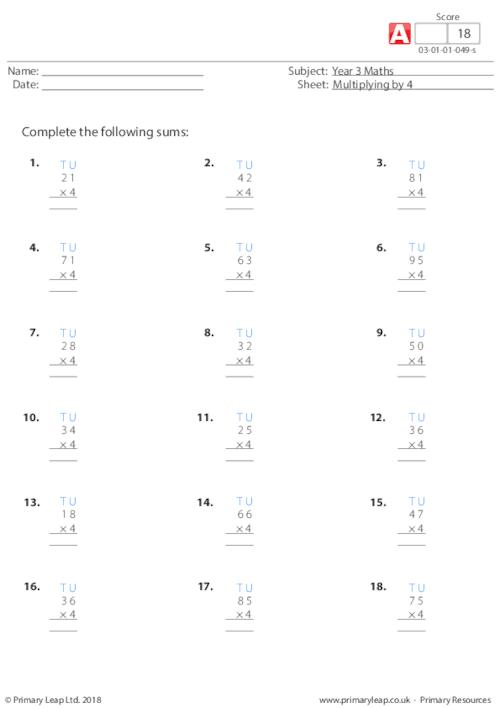of 2We have hundreds of year 3 maths worksheets to help your child feel more confident in their own abilities and continue to develop their understanding of numbers. Our maths worksheets will help your child learn more about fractions, including tenths, find perimeters of 2D shapes, recognise angles, use bar charts, and much more.Learn

One of the best things about printable worksheets is that they make learning fun and easy. Explore our educational resources based on Year 3 Maths and keep your children learning through these fun and engaging exercises.Progress

Our Year 3 Maths printable worksheets are designed for progressive learning. Our engaging content enables a child to learn at their own speed and gain confidence quickly. When we help a child believe in themselves, we empower them for life.Improve

Printable worksheets are a great way to enhance creativity and improve a child’s knowledge. Help your child learn more about Year 3 Maths with this great selection of worksheets designed to help children succeed.